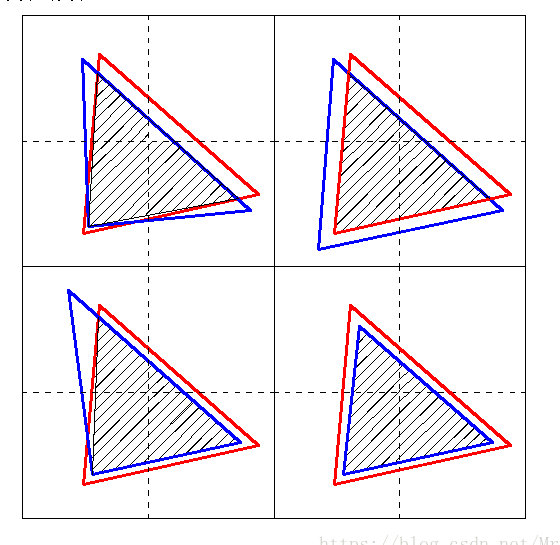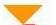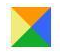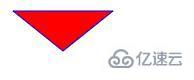• 共有10个三角形背景图片，要求把10个背景图片组合成一大的六边形背景图片 每小的三角形背景图片正中间有文字显示，且这些文字是动态变化的（文字来源——数据库中查询所得） 求解，多谢。联系qq:787457074net
• 【OpenGL】三、OpenGL 绘制三角形 ( 绘制单个三角形 | 三角形绘制顺序 | 绘制多个三角形 ) https://hanshuliang.blog.csdn.net/article/details/112796656 博客源码快照OpenGL
• 7-10 三角形 (100 分) 输入三条线段的长度（整型），根据是否能组成三角形，输出以下结果之一：锐角三角形、直角三解形、钝角三角形、不能构成三角形。 输入格式: 三整数 输出格式: 锐角三角形输出：Acute ...
7-10 三角形 (100 分)
输入三条线段的长度（整型），根据是否能组成三角形，输出以下结果之一：锐角三角形、直角三解形、钝角三角形、不能构成三角形。
输入格式:
三个整数
输出格式:
锐角三角形输出：Acute triangle
直角三解形输出：Right triangle
钝角三角形输出：Obtuse triangle
不能构成三角形输出：Not triangle
输入样例:
2 3 3
输出样例:
Acute triangle
输入样例:
5 4 3
输出样例:
Right triangle
输入样例:
6 4 3
输出样例:
Obtuse triangle
输入样例:
8 4 3
输出样例:
Not triangle
已知三边判断三角形
两边之和大于第三边且两边只差小于第三边可构成三角形
较小两边的平方和 == 最大边平方和 为直角三角形
较小两边的平方和 < 最大边平方和 为钝角三角形
较小两边的平方和 > 最大边平方和 为锐角
展开全文c语言
• 如图所示，两个三角形重合存在很多种情况，上图举出其中4例子，本文要讲的是已知两个三角形顶点坐标，求重叠部分最大三角形的顶点坐标； 虽然已知顶点坐标，位置关系已经确定，但是编程解决的是一类问题，...如图所示，两个三角形重合存在很多种情况，上图举出其中4个例子，本文要讲的是已知两个三角形三个顶点坐标，求重叠部分最大三角形的顶点坐标；
虽然已知顶点坐标，位置关系已经确定，但是编程解决的是一类问题，所以各种情况还是要考虑到。

定义变量保存结果
   double resultx;
double resulty;

两个三角形的坐标变量
	double coordinate-X;
double coordinate-Y;
double coordinate-XX;
double coordinate-YY;
coordinate-X=x1;//
coordinate-Y=y1;
coordinate-X=x2;
coordinate-Y=y2;
coordinate-X=x3;
coordinate-Y=y3;
coordinate-XX=PPx1;
coordinate-YY=PPy1;
coordinate-XX=PPx2;
coordinate-YY=PPy2;
coordinate-XX=PPx3;
coordinate-YY=PPy3;

第一步做的是判断有没有三角形的一个顶角是否在另一个三角形内部，如果有那么这个顶角一定是内部最大三角形的一个顶点

 std::vector<double> Nodeposy;
std::vector<double> Nodeposx;
//判断有没有一个三角形的顶点在另一个三角形的内部的情况（根据分析在三角形内部的顶点一定是重叠部分三角形的一个顶点）
//面积法判断
//S=(1/2)*|(x1y2+x2y3+x3y1-x1y3-x2y1-x3y2)|  //S=1/2|x1(y3-y2)+x2(y1-y3)+x3(y2-y1)|
double curs=abs(x1*(y2-y3)+x2*(y3-y1)+x3*(y1-y2));//计算三角形的面积，用的是向量
double pps=abs(PPx1*(PPy2-PPy3)+PPx2*(PPy3-PPy1)+PPx3*(PPy1-PPy2));//计算三角形的面积，用的是向量法
double sall=0.0f;

//当前三角是有有顶点在匹配色域空间中
for (int i=0;i<3;i++)
{
for (int n=0;n<3;n++)
{
for(int m=n+1;m<3;m++)
{
sall+=abs(coordinate-XX[n]*(coordinate-YY[m]-coordinate-Y[i])+coordinate-X[i]*(coordinate-YY[n]-coordinate-YY[m])+coordinate-XX[m]*(coordinate-Y[i]-coordinate-YY[n]));
}
}
if(abs(sall-pps)<0.01f)//面积相等说明在内
{
Nodeposy.push_back(coordinate-Y[i]);
Nodeposx.push_back(coordinate-X[i]);
}
sall=0.0f;
}

//两个三角形重合
if (Nodeposy.size()==3)
{
goto LLL;
}//当前三角是否有顶点在另一个三角中
sall=0.0f;
for (int j=0;j<3;j++)
{
for (int n=0;n<3;n++)
{
for(int m=n+1;m<3;m++)
{
sall+=abs(coordinate-X[n]*(coordinate-Y[m]-coordinate-YY[j])+coordinate-XX[j]*(coordinate-Y[n]-coordinate-Y[m])+coordinate-X[m]*(coordinate-YY[j]-coordinate-Y[n]));
}
}
if(abs(sall-curs)<0.01f)//面积相等说明在内
{
Nodeposy.push_back(coordinate-YY[j]);
Nodeposx.push_back(coordinate-XX[j]);
}
sall=0.0f;
}

if (Nodeposy.size()>=3)//有三个点在内部的时候，直接认为是公共三角形（不在计算交点）
{
for(int i=0;i<Nodeposx.size();i++)//先清除重合的点
{
for(int j=0;j<Nodeposx.size();j++)
{
if (Nodeposx[i]==Nodeposx[j])
{
Nodeposx.erase(Nodeposx.begin()+j);
Nodeposy.erase(Nodeposy.begin()+j);
}
}
}
for(int m=0;m<3;m++)
{
resultx[i]=Nodeposx[i];
resulty[i]=Nodeposy[i];
}
return true;
}

第二步计算两个三角形所有的交点

   for(int i=0;i<3;i++)//计算所有直线方程
{
if (i<2)
{
k[i]=(coordinate-Y[i+1]-coordinate-Y[i])/(coordinate-X[i+1]-coordinate-X[i]);
b[i]=coordinate-Y[i]-k[i]*coordinate-X[i];

pk[i]=(coordinate-YY[i+1]-coordinate-YY[i])/(coordinate-XX[i+1]-coordinate-XX[i]);
pb[i]=coordinate-YY[i]-k[i]*coordinate-XX[i];
}
else
{
k[i]=(coordinate-Y[i]-coordinate-Y)/(coordinate-X[i]-coordinate-X);
b[i]=coordinate-Y[i]-k[i]*coordinate-X[i];

pk[i]=(coordinate-YY[i]-coordinate-YY)/(coordinate-XX[i]-coordinate-XX);
pb[i]=coordinate-YY[i]-k[i]*coordinate-XX[i];
}
}

double curposy=0.0f;
double curposx=0.0f;
for(int i=0;i<3;i++)
{
for (int j=0;j<3;j++)
{
if (abs(k[i]-pk[j])>0.0f)//判断是否平行或重合
{
curposy=(b[i]-(k[i]*pb[j]/pk[j]))/(1.0f-(k[i]/pk[j]));
curposx=(pb[j]-b[i])/(k[i]-pk[j]);
//求线段坐标范围
int m,n;
if(i<2)m=i+1;
else m=0;
if(j<2)n=j+1;
else n=0;
double cux1min=coordinate-X[i]<coordinate-X[m]?coordinate-X[i]:coordinate-X[m]-0.5f;
double cux1max=coordinate-X[i]>coordinate-X[m]?coordinate-X[i]:coordinate-X[m]+0.5f;
double cuy1min=coordinate-Y[i]<coordinate-Y[m]?coordinate-Y[i]:coordinate-Y[m]-0.5f;
double cuy1max=coordinate-Y[i]>coordinate-Y[m]?coordinate-Y[i]:coordinate-Y[m]+0.5f;

double ppxmin=coordinate-XX[j]<coordinate-XX[n]?coordinate-XX[j]:coordinate-XX[n]-0.5f;
double ppxmax=coordinate-XX[j]>coordinate-XX[n]?coordinate-XX[j]:coordinate-XX[n]+0.5f;
double ppymin=coordinate-YY[j]<coordinate-YY[n]?coordinate-YY[j]:coordinate-YY[n]-0.5f;
double ppymax=coordinate-YY[j]>coordinate-YY[n]?coordinate-YY[j]:coordinate-YY[n]+0.5f;

//这里要取两根线最小的范围即为两根线的共有范围,所以小的里面取大的，大的里面取小的
double xmin=cux1min<ppxmin?ppxmin:cux1min;
double xmax=cux1max>ppxmax?ppxmax:cux1max;
double ymin=cuy1min<ppymin?ppymin:cuy1min;
double ymax=cuy1max>ppymax?ppymax:cuy1max;;

if(curposx>=xmin&&curposx<=xmax&&curposy>=ymin&&curposy<=ymax)
{
//将符合条件的交点记录
Nodeposx.push_back(curposx);
Nodeposy.push_back(curposy);
}
}
}
}

if (Nodeposx.size()<3)//计算到这仍然不到三个点说明计算错误返回false
{
return false;
}

第三步.找出面积最大的三角形

   std::vector<double> area;
std::vector<CString> index;
double s;
CString str;
for(int i=0;i<Nodeposx.size();i++)
{
for (int j=i+1;j<Nodeposx.size();j++)
{
for (int m=j+1;m<Nodeposx.size();m++)
{
s=abs(Nodeposx[i]*(Nodeposy[j]-Nodeposy[m])+Nodeposx[m]*(Nodeposy[i]-Nodeposy[j])+Nodeposx[j]*(Nodeposy[m]-Nodeposy[i]));
area.push_back(s);
str.Format("%i%i%i",i,j,m);
index.push_back(str);
}
}
}
double ss=0;
int num=0;
for (int n=0;n<area.size();n++)
{
if (area[n]>ss)
{
ss=area[n];
num=n;
}
}
int idx=atof(index[num]);
int anglenum;
anglenum=idx/100;
anglenum=idx%100/10;
anglenum=idx%10;

for(int m=0;m<3;m++)
{
resultx[i]=Nodeposx[anglenum[i]];
resulty[i]=Nodeposy[anglenum[i]];
}

index.clear();
area.clear();

Nodeposx.clear();
Nodeposy.clear();
return true;

至此最大重叠部分三角形的三个顶点就计算出来了

展开全文C++ 几何
• 利用css或html5画出一个三角形的方法发布时间：2020-09-14 14:49:22来源：亿速云阅读：83作者：小新这篇文章主要介绍利用css或html5画出一个三角形的方法，文中介绍的非常详细，具有一定的参考价值，感兴趣的小伙伴...

利用css或html5画出一个三角形的方法
发布时间：2020-09-14 14:49:22
来源：亿速云
阅读：83
作者：小新
这篇文章主要介绍利用css或html5画出一个三角形的方法，文中介绍的非常详细，具有一定的参考价值，感兴趣的小伙伴们一定要看完！
一、利用css的border属性，即可实现三角形的绘制
代码：border 属性--绘制三角形
.demo{
height:0;
width:0;
overflow: hidden;
font-size: 0;
line-height: 0;
border-color:#FF9600 transparent transparent transparent;
border-style:solid dashed dashed dashed;
border-width:20px;
}
效果图：利用css的border属性实现三角形的原理：css盒模型
一个盒子模型包括: margin+border+padding+content，上下左右边框交界处出呈现平滑的斜线. 利用这个特点, 通过设置不同的上下左右边框宽度或者颜色可以得到小三角, 小梯形等.调整宽度大小可以调节三角形形状..demo {
height:20px;
width:20px;
border-style:solid;
border-width:20px;
}
当把height和width都设置成0后，得到：把其他颜色都去掉，只留下橙色后，就得到一个三角形:二、利用html5的canvas画布，即可实现三角形的绘制canvas-绘制三角形
浏览器不支持canvas
var canvas=document.getElementById("canvas");//获取canvas对象
var ctx=canvas.getContext("2d"); //创建二维的绘图上下文对象
ctx.beginPath();
ctx.linewidth=20;
ctx.lineJoin="round"; //两条线交汇时的边角类型(miter 尖角默认 bevel斜角 round 圆角 )
ctx.moveTo(10,10);
ctx.lineTo(110,10);
ctx.lineTo(60,50);
ctx.closePath(); //closePath() 关闭路径 闭合
ctx.strokeStyle="blue";// strokeStyle 只能填充该路径的颜色
ctx.fillStyle="red";// fillStyle 填充字体颜色、填充路径区域、图形区域
ctx.fill();// fill() 填充字体
ctx.stroke();
}
效果图：利用html5的canvas画布，即可实现三角形绘制的重点:
三角形在画布中的三个坐标：moveTo(10,10)----左上角坐标，ctx.lineTo(110,10)-----右上角 坐标， ctx.lineTo(60,50)----下面坐标
以上是利用css或html5画出一个三角形的方法的所有内容，感谢各位的阅读！希望分享的内容对大家有帮助，更多相关知识，欢迎关注亿速云行业资讯频道！

展开全文• out了，老铁，来和我一起脑海畅想一正方形是由4等腰直角三角形构成，然后我想保留上边的三角形，那下边、左边、右边的三角形就没了(设置背景色transparent或者与背景相近的颜色)，最终结果就是一保留上边，...

本文将为您描述如何利用border书写三角形，建议考虑正方形,具体实现方法:网页做三角形图片，你还在拿ps调整吗？out了，老铁，来和我一起脑海畅想一个正方形是由4个等腰直角三角形构成，然后我想保留上边的三角形，那下边、左边、右边的三角形就没了(设置背景色transparent或者与背景相近的颜色)，最终结果就是一个保留上边，方向朝下的三角形，需要注意一点的是设置的css样式中width=0，如果不是就不能构成正方形，而是一个梯形；此外还可以通HTML实体里的三角形符号，朝上▲朝下▼朝左◄朝右►，最后通过font的大小颜色控制三角形！
网页做三角形图片，你还在拿ps调整吗？out了，老铁，来和我一起脑海畅想一个正方形是由4个等腰直角三角形构成，然后我想保留上边的三角形，那下边、左边、右边的三角形就没了(设置背景色transparent或者与背景相近的颜色)，最终结果就是一个保留上边，方向朝下的三角形，需要注意一点的是设置的css样式中width=0，如果不是就不能构成正方形，而是一个梯形；此外还可以通HTML实体里的三角形符号，朝上▲朝下▼朝左◄朝右►，最后通过font的大小颜色控制三角形！
1
2
3
4
5
6 怎样不用图片来做一个三角形图标
7
8 *{
9 margin: 0;
11 }
12 /* .box1,.box2,.box3,.box4{
13 font-size: 66px;
14 color: lightcoral;
15 cursor: pointer;
16 } *//*HTML实体里的三角形符号*/
17 .box{
18 width: 0px;
19 border-left: 100px solid red;
20 border-right: 100px solid yellow;
21 border-top: 100px solid green;
22 border-bottom: 100px solid #000;
23 }/*四个三角形构成一个正方形，想要哪个边另外三边transparent*/
24 .box{
25 width: 0px;
26 border-left: 100px solid transparent;
27 border-right: 100px solid transparent;
28 border-top: 100px solid red;
29 border-bottom: 100px solid transparent;
30 }
31 .triangle1{
32 width: 0px;
33 border: 100px solid yellow;
34 border-top-color:transparent;
35 border-left-color: transparent;
36 border-right-color: transparent;
37 }
38 /*需要多大的三角形就设置相应像素的border值，然后将其中三个方向的边框颜色设置为透明或者将边框颜色设置为与背景相同的颜色即可*/
39
40
41
42
43

▲

44

▼

45

◄

46

►

47
48
49
50
51
52
如果想了解更多纯CSS写三角形-border法，建议参考下小平头~ 与mumu42(后续有时间再补全该页面border书写三角形的方法)如何利用border书写三角形，建议考虑正方形就为您介绍到这里，感谢您关注懒咪学编程c.lanmit.com.
本文地址：https://c.lanmit.com/Webqianduan/htmlyucss/45022.html

展开全文• 2、在找出三点不能组成三角形的情况；有斜率相等和不存在斜率两种情况； 完整代码如下： #include&lt;iostream&gt; #include &lt;vector&gt; #include &lt;assert.h&gt; using ...
• python的turtle库可以方便的绘制三角形。工具/原料python3及turtle库（自带，不用另外安装）方法/步骤1先画一条横线，再旋转120度，再画一条横线，再旋转120度，再画一条横线。这样三角形就画好了。算法就是这样。2...
• // 设置顺时针方向 CW : Clock Wind 顺时针方向 // 默认是 GL_CCW : Counter Clock Wind 逆时针方向 glFrontFace(GL_CW);shell
• 《软件测试三角形问题(覆盖测试)》由会员分享，可在线阅读，更多相关《软件测试三角形问题(覆盖测试)(8页珍藏版)》请在人人文库网上搜索。1、成绩辽宁工程技术大学上机实验报告课程名称软件测试与评估实验题目基于...
• 等等许多生活中常见的三角形例子，学习三角形，你就会明白“三角形具有稳定性”的原理。下面有位老师-Andy老师给大家整理了一些有需要完整电子打印版的私信三角形知识点总结一、 基础知识1、三角形的定义： 由不在...
• 作者 | 李银城三角形的场景很常见，打开一页面可以看到各种各样的三角形：由于div一般是四边形，要画个三角形并不是那么直观。你可以贴一张png，但是这种办法有点low，...
• ## 数字三角形

万次阅读 2021-02-28 20:27:19
打印数字三角形,从1开始输出，第i行输出i数，每数字按4位置输出 注：c语言中 %4d可以输出一数，占据四位置，右对齐。 输入描述: 输入一行，包含一整数n 1 <= n <= 1000 输出描述: 输出n行，第i行...c语言 c++ 字符串
• 1. 通过向量定义三角形 ...这三线段，共同组成了一个三角形，定义集合S包含三线段： 集合S中的所有位置向量的终点构成了一个三角形 2. 定义变换矩阵 假设线性变换为： 那么，A就称为变换矩阵，例如： ...
• 题目：输入三角形3条边的长度值(均为正整数)，判断是否能为直角三角形的3边长。如果可以，则输出 yes，如果不能，则输出 no。如果根本无法构成三角形，则输出not a triangle。思路：先判断3条边能否构成普通三角形...
• 设计一个三角形类Triangle,包含三角形三条边长的私有数据成员，另有一重载运算符“+",以实现求两个三角形对象的面积之和，进一步实现3或更多的三角形对象相加的面积之和。 分析提示：在Triangle类中设计一友元...c++ 多态
• 北京市2019年中考数学复习三角形课时训练二直角三角形与勾股定理
• 个三角形的时候，再加一个三角形，每一条变会与第一个三角形的两条边相交，这样增加2三角形，即两面。f(2)=3*2+f(1)，再加一个三角形，每一条边会与前两个三角形的四条边相交，形成四三角形,f(3)=3*4+f...
• 在主程序中定义一TRI类的对象test，从键盘输入三角形3顶点的坐标值，如：(10，0)、(50，0)、(100，100)，调用data完成赋值，并用process计算，最后调用show函数输出三角形面积值。然后按上述要求完成对该类的...
• CSS是一很神奇的东西，在我们的前端开发中用到很多，那么怎么用CSS实现一个三角形呢？工具/原料前端开发工具方法/步骤我们的思路是使用border边框来实现三角形的样式，因为border的边框是由四个三角形组成的。首先......